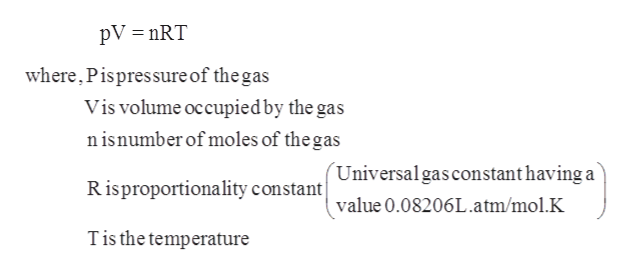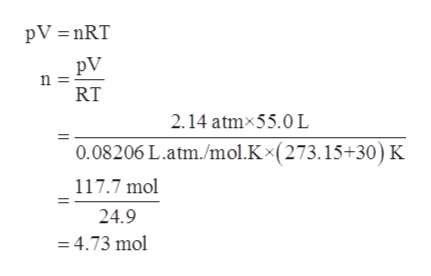# What is the mole fraction of NO in a 55.0-L gas cylinder at 30°C which comes from a mixture of N2and NO if the cylinder contains 3.238 mol of N2 at a total pressure of 2.14 atm?

Question
6 views

What is the mole fraction of NO in a 55.0-L gas cylinder at 30°C which comes from a mixture of N2and NO if the cylinder contains 3.238 mol of N2 at a total pressure of 2.14 atm?

check_circle

Step 1

Given information:

Volume of gas cylinder = 55.0 L

Temperature = 30 °C

Moles of nitrogen gas = 3.238 mol

Total pressure = 2.14 atm

Step 2

Ideal gas law gives the empirical relationship between pressure, volume, temperature, and amount of gas that is number of moles of a gas. The ideal gas equation is represented as follows:help_outlineImage TranscriptionclosepV = nRT where, Pispressure of the gas Vis volume occupied by the gas n isnumber of moles of the gas (Universalgasconstant having a Risproportionality constant value 0.08206L.atm/mol.K Tis the temperature fullscreen
Step 3

Using ideal gas equation first total moles o...help_outlineImage TranscriptionclosepV =nRT pV n = RT 2.14 atmx55.0 L 0.08206 L.atm./mol.K×(273.15+30) K 117.7 mol 24.9 = 4.73 mol fullscreen

### Want to see the full answer?

See Solution

#### Want to see this answer and more?

Solutions are written by subject experts who are available 24/7. Questions are typically answered within 1 hour.*

See Solution
*Response times may vary by subject and question.
Tagged in

### Chemistry